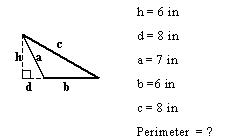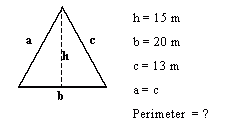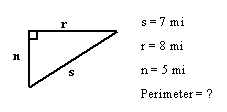Name: ___________________Date:___________________

 Email us to get an instant 20% discount on highly effective K-12 Math & English kwizNET Programs!

Grade 7 - Mathematics9.35 Perimeter of Triangle - Using Pictures

 Perimeter of Triangle = side + side + side Example 1: Find the perimeter of the right angle triangle.Perimeter = 4 + 5 +5 = 14 in Example 2: Find the perimeter of the right angle triangle.Perimeter = 5 + 6 +4 = 15 cm Example 3: Find the perimeter of the acute angle triangle.Perimeter = 13 + 13 + 16 = 42 m Example 4: Find the perimeter of the obtuse angle triangle.Perimeter = 7 + 6 + 8 = 21 in Directions: Find the perimeter of the triangles. Write the answers without the units.
 Q 1: Find the perimeter of the triangle.Answer: Q 2: Find the perimeter of the triangle.Answer: Q 3: Find the perimeter of the triangle.Answer: Q 4: Find the perimeter of the triangle.Answer: Q 5: Find the perimeter of the triangle.Answer: Q 6: Find the perimeter of the triangle.Answer: Question 7: This question is available to subscribers only! Question 8: This question is available to subscribers only!